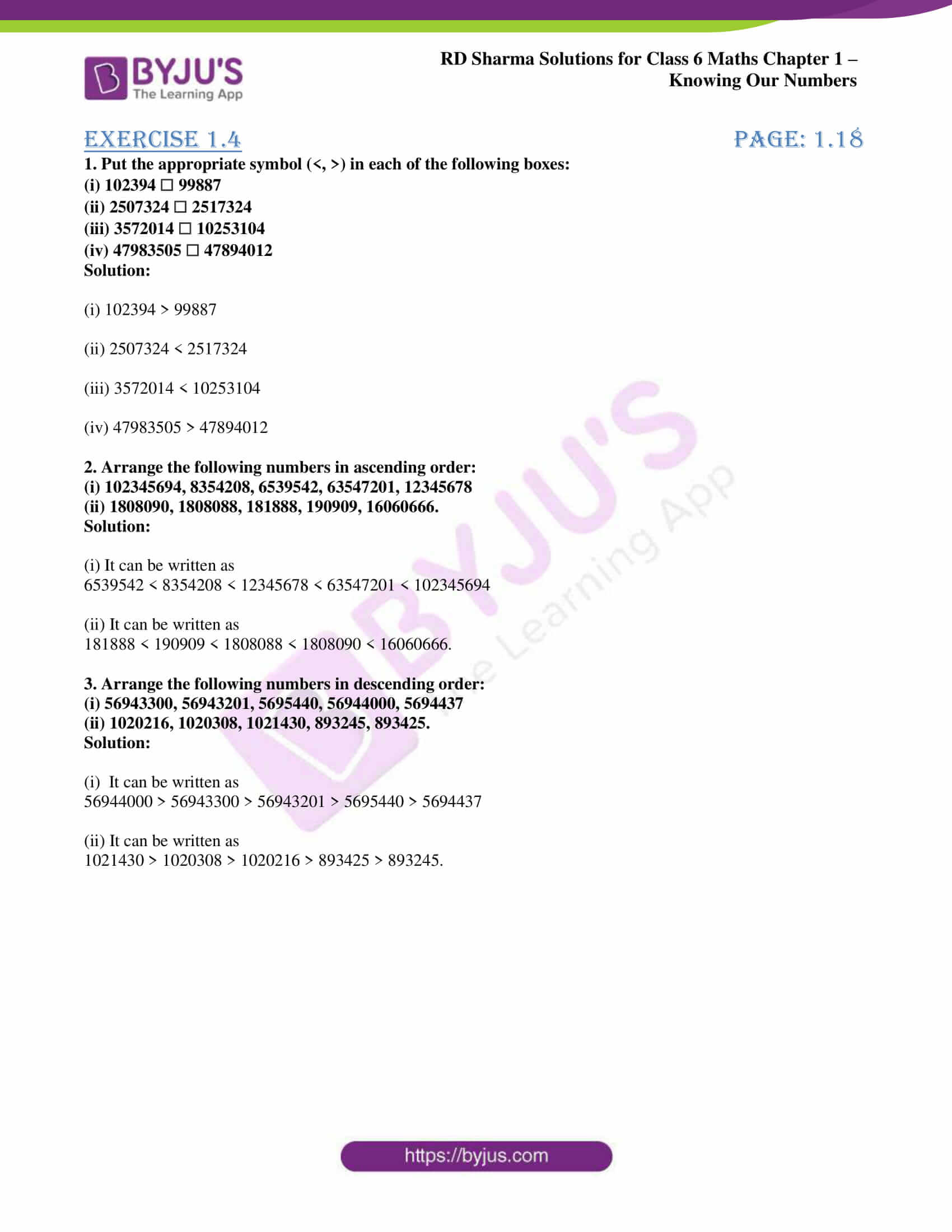# RD Sharma Solutions for Class 6 Maths Chapter 1: Knowing Our Numbers Exercise 1.4

## RD Sharma Solutions for Class 6 Maths Exercise 1.4 PDF

Class 6 is an important phase in a student’s life as it helps them to learn basic concepts which will help in solving problems in higher grades. Once the student understands the basics in Mathematics, solving problems becomes further easy. In order to help students, faculty at BYJU’S has created exercise wise problems which help in gaining knowledge. The students can solve the problems using PDF of solutions which help in clearing the doubts by themselves. RD Sharma Solutions for Class 6 Maths Chapter 1 Knowing Our Numbers Exercise 1.4 are provided here.

## RD Sharma Solutions for Class 6 Maths Chapter 1: Knowing Our Numbers Exercise 1.4 Download PDF## Access answers to Maths RD Sharma Solutions for Class 6 Chapter 1: Knowing Our Numbers Exercise 1.4

#### Exercise 1.4 page: 1.18

1. Put the appropriate symbol (<, >) in each of the following boxes:

(i) 102394 ☐ 99887

(ii) 2507324 ☐ 2517324

(iii) 3572014 ☐ 10253104

(iv) 47983505 ☐ 47894012

Solution:

(i) 102394 > 99887

(ii) 2507324 < 2517324

(iii) 3572014 < 10253104

(iv) 47983505 > 47894012

2. Arrange the following numbers in ascending order:

(i) 102345694, 8354208, 6539542, 63547201, 12345678

(ii) 1808090, 1808088, 181888, 190909, 16060666.

Solution:

(i) It can be written as

6539542 < 8354208 < 12345678 < 63547201 < 102345694

(ii) It can be written as

181888 < 190909 < 1808088 < 1808090 < 16060666.

3. Arrange the following numbers in descending order:

(i) 56943300, 56943201, 5695440, 56944000, 5694437

(ii) 1020216, 1020308, 1021430, 893245, 893425.

Solution:

(i) It can be written as

56944000 > 56943300 > 56943201 > 5695440 > 5694437

(ii) It can be written as

1021430 > 1020308 > 1020216 > 893425 > 893245.

### RD Sharma Solutions Class 6 Maths Chapter 1 – Knowing Our Numbers Exercise 1.4

RD Sharma Solutions Class 6 Maths Chapter 1 Knowing Our Numbers Exercise 1.4 helps us understand the method of comparing numbers based on which is the greatest and which is the smallest in the exercise given.

### Key features of RD Sharma Solutions for Class 6 Maths Chapter 1: Knowing Our Numbers Exercise 1.4

• The students improve their logical thinking and analytical abilities by using the solutions created by experts.
• PDF can be downloaded easily by the students either chapter wise or exercise wise which is time saving.
• Students who are unable to understand concepts during class hours can understand the concepts and methods by using RD Sharma Solutions.
• It is a vital resource for revision purpose to understand the concepts which are of greater importance.